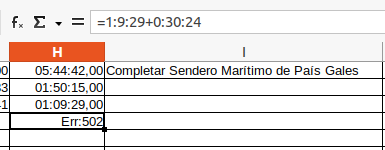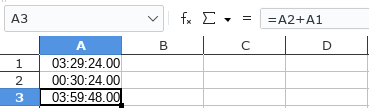# Adding to a time field

Hi,

I have a time field in the format [HH]:MM:SS,00 which represents the length of time I’ve run so far in the week. So every day, after I’ve been for a run, I’d like to select the cell for the weekly running total and add the new time. For example if I’ve so far run for 03:29:24,00 and I’ve just come in from a run that lasted for 00:30:24,00 I’d like to be able to edit that time by just adding the new one: 03:29:24,00 + 00:30:24,00, However if I try to do this, I just get a 502 error.Note: I am not trying to add two cells, but rather update the data in a single cell to reflect the new time spent running during the current week by adding the new amount of time.

Note also: the formula in the image above is its form after leaving the cell, so leading and trailing zeroes have been removed (tens of hours, tens of minutes and hundredths of a second).

Is there a way to do this without causing an error?

Thanks

Peter

In general that works, hence your error is within details of you sheet. So please upload a sample, which shows your solution not working.Hello,

you seem to literally insert into a cell `=03:29:24,00 + 00:30:24,00`. This must fail.

`03:29:24,00` is a text which needs to be converted by Calc to a number during input to be a real Calc time (A Calc time or a time duration is a fraction of a day, e.g. 0,5 is 12:00 hours, 0,75 is 18:00 hours an 1,5 is 36 hours). Now you are trying to use an add operation using operator `+`, but you still have the text values, which are not yet converted to a number. But a `+` requires numerical data. Hence you get error 502 Invalid argument. Putting the values into cells turns the textual input into numbers (by some input recognition algorithm) and using `+` in `=A1+A2` is correct since now both operands left and right of the plus sign are decimal numbers.

Solution:

• Add one time (duration) into one cell (let’s say `A1`)
• Add the next time (duration) into another cell (let’s say `A2`)
• Assure both data being correctly converted to a number during input (blue colored value, if using `View -> Value Highlighting`)
• Add `=A1+A2` into a third cell to add both times.

Hope that helps.

Hmm. I assumed the cell formatting would take care of that, because that’s what happens with the number field to the left (i.e. the distance run), but obviously not. It’s rather strange, though: if I enter a time like 69:00,00 it is automatically converted to 1:09:00,00 - so it does know that it’s dealing with a time.

Many thanks for your input, but the solution is, unfortunately, more work than just doing the calculation in my head, which is what I do at the moment.

Peter

Sorry - but then I wonder why you are using a spreadsheet application at all.

Hey! No need to apologise. I’m grateful for your help.

I decided to use a spreadsheet because I thought I would be able to do the addition in the sheet and not in my head, but it seems I was wrong. You live and learnIn formula expressions `:` is the cell range operator, so it doesn’t do what you think it does. Using explicit time cells is the spreadsheet way to calculate with times.

OK. So does this mean, as it seems to, that you can’t add up two explicit time values? For example, is there not a way to convert the explicit values to time values (which are strings, apparently), add them, and then convert them back? Something like

string(time(‘nn:nn:nn’) + time(‘nn:nn:nn’))

I’ve no idea if those functions exist or, if they did, if they would work in this way.

OK. So does this mean, as it seems to, that you can’t add up two explicit time values?

You obviously did not understand my answer: 03:29:24,00 is not a calc time value when used before it is converted to a time value through input recognition. If you use `=` you indicate that you are about to enter a formula and somehow disable conversion of 03:29:24,00 into a time value.

string(time(‘nn:nn:nn’) + time(‘nn:nn:nn’))
I’ve no idea if those functions exist or, if they did, if they would work in this way.

TIME() exists, see help.

You don’t need that complexity; this works:

``="03:29:24"+"00:30:24"``
1 Like

Only if Tools → Options → Calc → Formula, Detailed Calculation Settings isn’t set to `Generate #VALUE! error` nor to `Treat as zero` nor to `Convert also locale dependent` and the locale doesn’t use `:` colon as time separator…